### Computational Details

In the computation of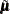and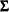described in the previous section, the inverse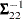is never actually computed; an equation of the form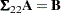is solved for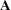by using a modified Gaussian elimination algorithm that takes advantage of the fact that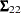is symmetric with constant diagonal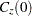that is larger than all off-diagonal elements. The SINGULAR= option pertains to this algorithm. The value specified for the SINGULAR= option is scaled bybefore comparison with the pivot element.

#### Memory Usage

For conditional simulations, the largest matrix held in core memory at any one time depends on the number of grid points and data points. Using the previous notation, the data-data covariance matrix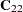is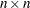, whereis the number of nonmissing observations for the VAR= variable in the DATA= data set. The grid-data cross covariance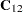is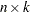, whereis the number of grid points. The grid-grid covariance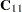is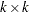. The maximum memory required at any one time for storing these matrices is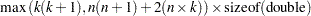There are additional memory requirements that add to the total memory usage, but usually these matrix calculations dominate, especially when the number of grid points is large.